## Example Questions

### Example Question #1 : How To Find The Reciprocal Of A Fraction

What is the reciprocal of the following fraction: 18/27

9

-18/27

27/18

9/27

27/18

Explanation:

A fraction multiplied by its reciprocal will equal 1. To find the reciprocal of a fraction, switch the denominator and numerator. The reciprocal of 18/27 is 27/18.

### Example Question #1 : How To Find The Reciprocal Of A Fraction

What is the reciprocal of the fraction below?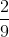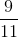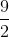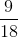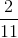Explanation:

The reciprocal of a fraction can be obtained by switching the numerator and denominator.

The numerator in this case isso that will become the denominator.

The denominator in this case isso that will become the numerator.

Threfore the reciprocal ofis.

### Example Question #3 : How To Find The Reciprocal Of A Fraction

What is the reciprocal of?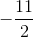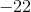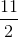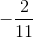Explanation:

The reciprocal can be determined by taking one dividing the entire quantity.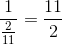This is just flipping the numerator and the denominator.

The answer is.

### Example Question #1 : How To Find The Reciprocal Of A Fraction

What is the reciprocal of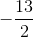?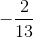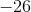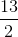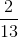Explanation:

To find the reciprocal, take 1 and divide by the entire quantity of the fraction. The reciprocal is simply swapping the placement of the numerator and the denominator.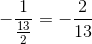### Example Question #1 : How To Find The Reciprocal Of A Fraction

What is the negative reciprocal of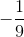?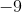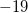Explanation:

The negative reciprocal of a number is to take negative one, and divide by the value.  Simply swap the numerator and denominator and add a negative sign.

Recall that dividing by a fraction is the same as multiplying by the reciprocal.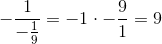The correct answer is:### Example Question #1 : Reciprocals

Find the reciprocal of the fraction: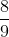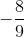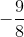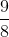Explanation:

The reciprocal of a fraction is one over the quantity of the fraction.Simply switch the terms of the numerator and denominator.

The answer is.

### Example Question #1 : How To Find The Reciprocal Of A Fraction

The reciprocal ofis equal to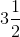.

What is the reciprocal of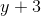?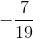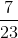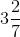Explanation:

The reciprocal ofis equal to, so- which is the reciprocal of the reciprocal of- is the reciprocal of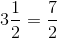, sois the reciprocal of this, or.

Since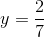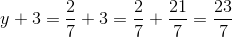The reciprocal ofis.

### All SAT Math Resources